RRB JE CE (CBT I) Mock Test- 1

# RRB JE CE (CBT I) Mock Test- 1

Test Description

## 100 Questions MCQ Test RRB JE Mock Test Series for Civil Engineering (CE) | RRB JE CE (CBT I) Mock Test- 1

RRB JE CE (CBT I) Mock Test- 1 for Railways 2023 is part of RRB JE Mock Test Series for Civil Engineering (CE) preparation. The RRB JE CE (CBT I) Mock Test- 1 questions and answers have been prepared according to the Railways exam syllabus.The RRB JE CE (CBT I) Mock Test- 1 MCQs are made for Railways 2023 Exam. Find important definitions, questions, notes, meanings, examples, exercises, MCQs and online tests for RRB JE CE (CBT I) Mock Test- 1 below.
Solutions of RRB JE CE (CBT I) Mock Test- 1 questions in English are available as part of our RRB JE Mock Test Series for Civil Engineering (CE) for Railways & RRB JE CE (CBT I) Mock Test- 1 solutions in Hindi for RRB JE Mock Test Series for Civil Engineering (CE) course. Download more important topics, notes, lectures and mock test series for Railways Exam by signing up for free. Attempt RRB JE CE (CBT I) Mock Test- 1 | 100 questions in 90 minutes | Mock test for Railways preparation | Free important questions MCQ to study RRB JE Mock Test Series for Civil Engineering (CE) for Railways Exam | Download free PDF with solutions
 1 Crore+ students have signed up on EduRev. Have you?
RRB JE CE (CBT I) Mock Test- 1 - Question 1

### Two trains 165 m and 175 m long run at the speed of 50 km/h and 52 km/h respectively in opposite directions on parallel tracks. What is the time (in seconds) they take to cross each other?

Detailed Solution for RRB JE CE (CBT I) Mock Test- 1 - Question 1 Relative speed = (50 + 52) km/h = (102 x 5/18) m/s = (255/9) m/s.

Distance covered in crossing each other = (165 + 175) = 340 m.

∴ Required time = (340 x 9/255) = 12 s.

RRB JE CE (CBT I) Mock Test- 1 - Question 2

### If two equations: 2x2 - Ax - 20 = 0 and 2x2 - 11x + 12 = 0 have one root in common, then what is the value of 'A' if the value of A is positive?

Detailed Solution for RRB JE CE (CBT I) Mock Test- 1 - Question 2 2x2 - 11x + 12 = 0

(2x - 3)(x - 4) = 0

x = 1.5 and 4

Since both the equations have one root in common-

Case 1: When x = 1.5 is common-

2(1.5)2 - A(1.5) - 20 = 0

RRB JE CE (CBT I) Mock Test- 1 - Question 3

### Divya has 3 types of coins having denomination of 20p, 10p and 5p. Their numbers are in the ratio of 1: 2 : 3. If Divya has total money of Rs. 44 then how many 5p coins are there?

Detailed Solution for RRB JE CE (CBT I) Mock Test- 1 - Question 3 Since the ratio between the numbers of coins of 20p, 10p and 5p is 1 : 2 : 3 So let us assume the number of 20p coins = a

Then number of 10p coins is 2a and number of 5p coins is 3a.

Total worth of 20p coins = number of coins X worth of each coin = a X (1/5) = Rs (a/5)

Total worth of 10p coins = 2a X (1/10) = Rs (a/5)

Similarly total worth of 5p coins = 3a X (1/20) = Rs (3a/20)

Since total money with the Divya is Rs 44 hence

a/5 + a/5 + 3a/20 = 44 => 2a/5 + 3a/20 = 44

=> 8a/20 + 3a/20=44 => 11a/20 =44 => a = 80

So the number of 5p coins = 3a = 80 x 3 = 240

RRB JE CE (CBT I) Mock Test- 1 - Question 4

The difference between 42% and 23% of a number is 304. What is 72% of that number?

Detailed Solution for RRB JE CE (CBT I) Mock Test- 1 - Question 4 Let the number be 100x.

∴ 42x - 23x = 304

=> 19x = 304

=> x = 16

∴ The number is 100 x 16 = 1600.

=> 72% of the number = 0.72 x 1600 = 1152

RRB JE CE (CBT I) Mock Test- 1 - Question 5

What is the value of 60 ÷ [{21 ÷ 7 x √(√625 + √121)} − 6] x 5 - 42 ÷ 3 x 2 = ?

Detailed Solution for RRB JE CE (CBT I) Mock Test- 1 - Question 5 60 ÷ [{21 ÷ 7 x √(√625 + √121)} − 6] x 5 - 42 ÷ 3 x 2

= 60 ÷ [{3 x √(25 + 11)} − 6] x 5 - 14 x 2

= 60 ÷ [{3 x √(36)} − 6] x 5 - 28

= 60 ÷ [{3 x 6} − 6] x 5 - 28

= 60 ÷ [18 − 6] x 5 - 28

= 60 ÷ 12 x 5 - 28

= 5 x 5 - 28

= -3

RRB JE CE (CBT I) Mock Test- 1 - Question 6

Three pipes x, y and z can fill a water tanker in 20, 25 and 30 minutes respectively. All the three pipes are opened to fill the tanker and if the pipe y is closed 2 minutes before the water tanker gets filled, then in how much time will the tank get filled?

Detailed Solution for RRB JE CE (CBT I) Mock Test- 1 - Question 6 Let the time after which the water tanker gets filled be 't' minutes.

In one minute pipe x can fill 1/20 part.

In t minutes, pipe x can then fill t/20 part.

Therefore, t/20 + t-2/25 + t/30 = 1

On solving, we get t = 324/37 minutes.

RRB JE CE (CBT I) Mock Test- 1 - Question 7

If [4x - (1/12x) = 8], then what is the value of [x2 + {1/(2304x2)}] ?

Detailed Solution for RRB JE CE (CBT I) Mock Test- 1 - Question 7 4x - 1/12x = 8

=> 4(x - 1/48x) = 8

=> x - 1/48x = 2 ------ (i)

Squaring both sides, we get

(x - 1/48x)2 = 4

=> x2 + 1/(2304x2) - 2x/48x = 4 ------ [(a - b)2 = a2 + b2 - 2ab]

x2 + 1/2304 x2 = 4 + 1/24 = 97/24

RRB JE CE (CBT I) Mock Test- 1 - Question 8

A boy throws a ball of mass 4 kg vertically downwards for a height of 20 metres. What is the velocity of the ball which touches the ground? (Take g= 10 m/s2)

Detailed Solution for RRB JE CE (CBT I) Mock Test- 1 - Question 8 According to question,

Mass of ball = m = 4 kg, elevation from ground= h= 20 m; v= velocity at touch point

So, mgh = ½ (mv2)

Gives v= 20 m/s

mg = 1/2 (mv2)

2gh = v2

v2 = 2 x 10 x 20 = 400

v = 20 m/sec

RRB JE CE (CBT I) Mock Test- 1 - Question 9

Express 0.78414141.. into (p/q) form.

Detailed Solution for RRB JE CE (CBT I) Mock Test- 1 - Question 9 Let x = 0.78414141..

=> 100x = 78.414141.. (I)

=> 10000x = 7841.414141.. (II)

(II) - (I) gives,

9900x = 7763

=> x = 7763/9900

RRB JE CE (CBT I) Mock Test- 1 - Question 10

Nidhi borrowed Rs. 10000 from a bank for a period of two years at the rate of 10% per annum. From that amount, she invested Rs. 3500 in another bank which offers simple interest @12.5% per annum and remaining amount she invested into a chit fund which offers compound interest of 10% per annum. What is the profit obtained by Nidhi at the end of two years?

Detailed Solution for RRB JE CE (CBT I) Mock Test- 1 - Question 10 Total interest that Nidhi has to pay after 2 years = Rs. (10000 x 2 x 10)/100 = Rs. 2000

Interest obtained by Nidhi from the bank = Rs. (3500 x 2 x 12.5)/100 = Rs. 875

Interest obtained by Nidhi from Chit fund = 6500(1 + 0.1)2 - 6500 = Rs. 1365

So total interest obtained by Nidhi = Rs. (875 + 1365) = Rs. 2240

Profit = Rs. (2240 - 2000) = Rs. 240

RRB JE CE (CBT I) Mock Test- 1 - Question 11

A book was marked initially at Rs. 500 which A purchased at 8% discount. He marked up the price by 15% on his purchased price and sold after Rs. 20 discount to B. B marked up the price by 20% above his purchased price and sold to C after allowing Rs. 50.9 discount. What is the percent profit gained by B when sold to C?

Given below are the steps involved. Arrange them in the sequential order.

(A) Initial marked price of book = Rs. 500 and purchased price for A = 92% of 500 = Rs. 460

(B) Purchased price of book by B = 529 - 20 = Rs. 509

(C) Per cent profit = [(559.9 - 509)/509] x 100 = 10%

(D) Marked price of book by A = 115% of 460 = Rs. 529

(E) Marked price of book by B = 120% of 509 = Rs. 610.8 and purchased price of book by C = 610.8 - 50.9 = Rs. 559.9

Detailed Solution for RRB JE CE (CBT I) Mock Test- 1 - Question 11 The correct order is

(A) Initial marked price of book = Rs. 500 and purchased price for A = 92% of 500 = Rs. 460

(D) Marked price of book by A = 115% of 460 = Rs. 529

(B) Purchased price of book by B = 529 - 20 = Rs. 509

(E) Marked price of book by B = 120% of 509 = Rs. 610.8 and purchased price of book by C = 610.8 - 50.9 = Rs. 559.9

(C) Per cent profit = [(559.9 - 509)/509] x 100 = 10%

RRB JE CE (CBT I) Mock Test- 1 - Question 12

Seema purchased an item for Rs.9,600 and sold it for a loss of 5 percent. From that money she purchased another item and sold it for a gain of 5 percent. What is her overall gain/ loss?

Detailed Solution for RRB JE CE (CBT I) Mock Test- 1 - Question 12

SP = 9600 x 95 / 100 = Rs. 9,120

Second S.P. = 9120 x 100 / 100 = Rs. 9,576

Loss = 9600 - 9576 = 24

RRB JE CE (CBT I) Mock Test- 1 - Question 13

What is the angle between the hands of a clock at 8:30 a.m.?

Detailed Solution for RRB JE CE (CBT I) Mock Test- 1 - Question 13 At 8:30 a.m. minute hand points at 6 and and hour hand points exactly between 8 and 9.

So these two hands are apart by 2.5 blocks.

We know that the difference of angle of one block is 30°.

Hence the angle between the hands = 2.5 X 30° = 75°

RRB JE CE (CBT I) Mock Test- 1 - Question 14

Following data given below shows the sex ratio of India in last 10 years. Find the mode of sex ratio in India.

976, 979, 1084, 993, 1084, 993, 935, 979, 991, 979

Detailed Solution for RRB JE CE (CBT I) Mock Test- 1 - Question 14 The mode is a statistical term that refers to the most frequently occurring number found in a set of numbers.

Since, 979 is occurring frequently and number of occurrence of 979 is 3.

Hence mode of sex ratio in India in last 10 years is 979.

RRB JE CE (CBT I) Mock Test- 1 - Question 15

If 7x5 - 2x4 + 3x3 - 4x2 + 6x + 2k is divisible by (x + 1), then what is the value of k?

Detailed Solution for RRB JE CE (CBT I) Mock Test- 1 - Question 15 Since, 7x5 - 2x4 + 3x3 - 4x2 + 6x + 2k is divisible by (x + 1)

=> x = -1 must satisfy the given equation

=> 7(-1)5 - 2(-1)4 + 3(-1)3 - 4(-1)2 + 6(-1) + 2k = 0

=> -7 - 2 - 3 - 4 - 6 + 2k = 0

=> 2k = 22

=> k = 11

RRB JE CE (CBT I) Mock Test- 1 - Question 16

The ratio of two numbers is 7:5 and their H.C.F. is 6. What is their L.C.M.?

Detailed Solution for RRB JE CE (CBT I) Mock Test- 1 - Question 16 Let the numbers be 7x and 5x.

as, 7 and 5 are coprime pairs.

∴ Their H.C.F. = x = 6 (given).

=> The numbers are 42 and 30.

L.C.M. of 42 and 30 = 210.

RRB JE CE (CBT I) Mock Test- 1 - Question 17

From an external point O, a secant is drawn on a circle which meets the circle at points A and B respectively, if OA = 4 cm and AB = 60 cm. The length of the tangent drawn on circle from point O is (in cm)

Detailed Solution for RRB JE CE (CBT I) Mock Test- 1 - Question 17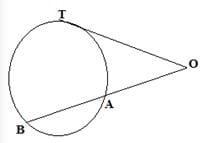Let the tangent be T

OT2 = OA.OB

=> OA = 4

=> OB = OA + AB

=> 4+60

=> 64

OT2 = 4x64

=> 256

=> OT = 16 cm

RRB JE CE (CBT I) Mock Test- 1 - Question 18

A copper-wire when bent in the form of a square enclose an area of 121 cm2. If the same wire is bent in the form of a circle, then the area of the circle is

Detailed Solution for RRB JE CE (CBT I) Mock Test- 1 - Question 18 Side of square = √121 = 11 cm

Length of wire = circumference of circle.

44 = 2 π r or r = (44 x 7)/(2 x 22) = 7 cm

Hence, area of circle = πr2 = 22/7 x 7 x 7 = 154 cm2

RRB JE CE (CBT I) Mock Test- 1 - Question 19

Table given below shows the total number of employees of four different grades in a department and average age of employees in those difference grades.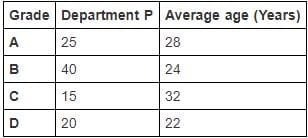Table given below shows the total number of employees of four different grades in a department and average age of employees in those difference grades.

Detailed Solution for RRB JE CE (CBT I) Mock Test- 1 - Question 19 Sum of ages of all employees in grade A = 25 x 28 = 700 years

Sum of ages of all employees in grade B = 40 x 24 = 960 years

Sum of ages of all employees in grade C = 15 x 32 = 480 years

Sum of ages of all employees in grade D = 20 x 22 = 440 years

Sum of ages of all the employees of the department P = 700 + 960 + 480 + 440 = 2580 years

Total employees in department P = 25 + 40 + 15 + 20 = 100

Average age of the employees of department P = 2580/100 = 25.8 years

RRB JE CE (CBT I) Mock Test- 1 - Question 20

What will be the percentage profit after selling an article at a certain price if there is a loss of 12.5% when the article is sold at half of the previous selling price?

Detailed Solution for RRB JE CE (CBT I) Mock Test- 1 - Question 20 Suppose the previous selling price = Rs. x

Selling price = Rs. x/2

There is a loss of 12.5% when selling price is Rs. x/2.

Cost Price = x/2 x 100/(100-12.5)= (100 x)/175= 4x/7

Now, when selling price is Rs. x, % profit =[(x-(4x/7))/(4x/7)] x 100 = (3x/4x) x 100 = 75%

RRB JE CE (CBT I) Mock Test- 1 - Question 21

If α, 3α+6, and 71α are in arithmetic progression, then find the value of α.

Detailed Solution for RRB JE CE (CBT I) Mock Test- 1 - Question 21 α, 3α + 6, and 71α are in arithmetic progression.

=> 3α + 6 - α = 71α - 3α - 6 = common difference of the arithmetic progression.

=> 2α = 68α - 6 - 6

=> 66α = 12

=> α = 12/66 = 2/11

RRB JE CE (CBT I) Mock Test- 1 - Question 22

One-fourth of Lainey's savings in Kisan Vikas Patra is equal to one-fifth of her savings in Employee Provident Fund. How much has she saved in Employee Provident Fund, if she has a total savings of ₹ 180,000?

Detailed Solution for RRB JE CE (CBT I) Mock Test- 1 - Question 22 Let her savings in Employee Provident Fund be ₹ x.

∴ Her savings in Kisan Vikas Patra = ₹ (180,000 - x)

∴ (180,000 - x)/4 = x/5

=> 900,000 - 5x = 4x

=> x = ₹ 100,000

RRB JE CE (CBT I) Mock Test- 1 - Question 23

When 20% of 40% of half of a number 'm' is added to 3/4th of 20% of itself, then a second number is obtained which is 21 less than the 40% of m. What is the difference between first and second number?

Detailed Solution for RRB JE CE (CBT I) Mock Test- 1 - Question 23 First number = m

Second number = (40% of m ) - 21

According to the question:

20% of 40% of (m/2) + (3/4) x 20% of m = (40% of m) - 21

m = 100

Second number = (40% of m) - 21 = 40% of 100 - 21 = 19

Difference = 100 - 19 = 81

RRB JE CE (CBT I) Mock Test- 1 - Question 24

What will come in place of (?) in this equation?

125% of [{? + 200 ÷ (16 ÷ 2 x 4 x (1/8))} ÷ 7.5 x 8 ÷ (18 ÷ 3 - 50% of 4)] = ?

Detailed Solution for RRB JE CE (CBT I) Mock Test- 1 - Question 24 Let '?' be denoted by 'a'.

125% of [{a + 200 ÷ (16 ÷ 2 x 4 x (1/8))} ÷ 7.5 x 8 ÷ (18 ÷ 3 - 50% of 4)] = a

=> 125% of [{a + 200 ÷ (8 x 4 x (1/8))} ÷ 7.5 x 8 ÷ (6 - 2)] = a

=> 125% of [{a + 200 ÷ 4} ÷ 7.5 x 8 ÷ 4] = a

=> 125% of [{a + 50} ÷ 7.5 x 2] = a

=> 125% of 2(a + 50)/7.5 = a

=> (5/4) x 2(a + 50)/7.5 = a

=> a + 50 = 3a

=> a = 25

RRB JE CE (CBT I) Mock Test- 1 - Question 25

If cos (900 - θ)/cos (300 + θ) = 1, then the value of sin θ + cos 2θ

Detailed Solution for RRB JE CE (CBT I) Mock Test- 1 - Question 25 cos (900 - θ)/cos (300 + θ) = 1

sin θ = cos (300 + θ)

sin θ = sin (900 - 300 - θ)

θ = 900 - 300 - θ

θ = 300

= (sin θ + cos 2θ)

= sin 300 + cos 600

= 1/2 + 1/2

= 1

RRB JE CE (CBT I) Mock Test- 1 - Question 26

A fraction is such that if it is squared and then the numerator is divided by 2, while the denominator is increased by 25%, the new fraction thus obtained is 4 times the original fraction. What is the new fraction?

Detailed Solution for RRB JE CE (CBT I) Mock Test- 1 - Question 26 Let the original fraction be x/y

According to given condition,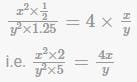RRB JE CE (CBT I) Mock Test- 1 - Question 27

What should come in place of question mark (?) in the following series?

15, 17, 23, 35, 55, (?)

Detailed Solution for RRB JE CE (CBT I) Mock Test- 1 - Question 27 15 + (1 x 2) = 17

17 + (2 x 3) = 23

23 + (3 x 4) = 35

35 + (4 x 5) = 55

55 + (5 x 6) = 85

RRB JE CE (CBT I) Mock Test- 1 - Question 28

How much does a watch lose per day, if its hands coincide every 65 minutes?

Detailed Solution for RRB JE CE (CBT I) Mock Test- 1 - Question 28 For a regular clock, we know that the hands of a watch meet after every 65(5/11) minutes.

Hence loss in 65 minutes = 65(5/11) - 65 = (5/11) minutes

Hence loss in 24 hours = (5 x 24 x 60) / (11 x 65) = 10(10/143) minutes

RRB JE CE (CBT I) Mock Test- 1 - Question 29

Fifteen years hence, the ratio of the ages of a man and his son will be 2:1. If the present age of the man is 45, then what is his son's present age?

Detailed Solution for RRB JE CE (CBT I) Mock Test- 1 - Question 29 Fifteen years hence the ration of age = 2:1.

Let the present age of the son be 'x'.

(45+15)/(x+15) = 2/1

2x + 30 = 60

x = 15

So, the present age of son is 15 years

RRB JE CE (CBT I) Mock Test- 1 - Question 30

Ramesh can do 20% more work as Mohan can do in same time. If Mohan can finish the work in 30 hours then Ramesh with the help of Mohan, can finish the same work in how many hours ?

Detailed Solution for RRB JE CE (CBT I) Mock Test- 1 - Question 30 Mohan can complete a work in 30 hours.

Ramesh can do 6/5 work in 30 hours.

So Ramesh can complete the work in 30 x (5/6) = 25 hours.

Together they can finish 1/30 + 1/25 of work in 1 hour, i.e., 11/150.

So in 150/11 hours, they will finish the work together.

RRB JE CE (CBT I) Mock Test- 1 - Question 31

In the following question, select the related letter/word/number from the given alternatives.

MUSIC: TUNE

Detailed Solution for RRB JE CE (CBT I) Mock Test- 1 - Question 31 As the tune is in music, rhythm is in dance.
RRB JE CE (CBT I) Mock Test- 1 - Question 32

Find out a similar set of the given set:

(64, 216, 125)

Detailed Solution for RRB JE CE (CBT I) Mock Test- 1 - Question 32 The given question set (64, 216, 125) is the perfect cube of (4, 6, 5). Similarly (8, 27, 64) is the perfect cube of (2, 3, 4).
RRB JE CE (CBT I) Mock Test- 1 - Question 33

Pointing to a boy Neha said, ‘He is the son of my grandfather’s only child. How is she related to that boy?

Detailed Solution for RRB JE CE (CBT I) Mock Test- 1 - Question 33 Grandfather's child is father, and son of a girl's father will be her brother.
RRB JE CE (CBT I) Mock Test- 1 - Question 34

In a code language, ‘S1357’ means ‘SWe are very happy,’ ‘S2639’ means ‘They are extremely lucky,’ and ‘S794’ means ‘SHappy and lucky.’ Which digit in that code language stands for ‘Svery’?

Detailed Solution for RRB JE CE (CBT I) Mock Test- 1 - Question 34 01357 → we are very happy .......(i)

2639 → they are extremely lucky ......(ii)

794 → happy and lucky ......(iii)

from (i) and (ii)

3 → are

from (ii) and (iii)

9 → lucky

from (i) and (iii)

7 → happy

from (iii)

4 → and

from (i)

1, 5 → we, very

Hence, we cannot find exact code for 'very'.

RRB JE CE (CBT I) Mock Test- 1 - Question 35

In a certain code language the word ‘FUTILE’ is written as ‘HYVMNI’. How will the word ‘PENCIL’ be written in that language?

Detailed Solution for RRB JE CE (CBT I) Mock Test- 1 - Question 35 Odd-placed letters are coded as two places forward and even-placed letters are coded as four places forward as in English alphabet.
RRB JE CE (CBT I) Mock Test- 1 - Question 36

Laxman start walking from his house and went 15 km to North, then he turned West and covered 10 km. Then he turned South and covered 5 km. Finally, turning to East, he covered 10 km. In which direction is he from his house?

Detailed Solution for RRB JE CE (CBT I) Mock Test- 1 - Question 36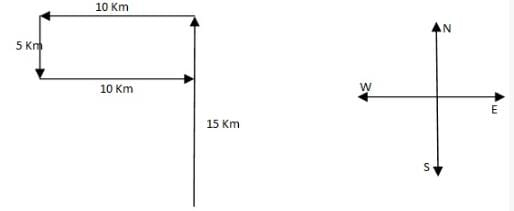From the figure, its clear that Laxman is in north of his house.

RRB JE CE (CBT I) Mock Test- 1 - Question 37

Given below are two Matrices of Twenty-five Cells, each containing two classes of alphabets. The columns and rows of Matrix I are numbered form 0 to 4 and that of Matrix II from 5 to 9. A letter from these Matrices can be represented first by its row number and next by its column number. In each of the following questions, identify one set of number pairs out of (1), (2), (3) and (4) which represents the given word.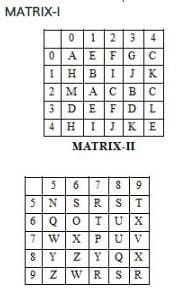RUST

Detailed Solution for RRB JE CE (CBT I) Mock Test- 1 - Question 37 RUST

R– 57, 97, 99

U– 68, 78

S– 56, 58, 98

T– 59, 67

Therefore, the correct answer is option (3) i.e. 97, 68, 56, 59

RRB JE CE (CBT I) Mock Test- 1 - Question 38

Arrange the following in a meaningful order:

1. Curd

2. Grass

3. Butter

4. Milk

5. Cow

Detailed Solution for RRB JE CE (CBT I) Mock Test- 1 - Question 38 Meaning ful order is

5. Cow

2. Grass

4. Milk

1. Curd

3. Butter

Hence option d is correct answer.

RRB JE CE (CBT I) Mock Test- 1 - Question 39

In the questions given below, some relationship has been expressed through symbols as shown below. Based on the meaning of these symbols and choose the correct answer.

ϕ means ‘less than.’

Δ means ‘not greater than.’

- means ‘equal to.’

+ means ‘not equal to.’

× means ‘not less than.’

= means ‘greater than.’

X ϕ Y - Z implies

Detailed Solution for RRB JE CE (CBT I) Mock Test- 1 - Question 39 Clearly, the meaning of the given symbols

X ϕ Y - Z ⇒ X < Y = Z

Using the proper notations/symbols in option (c), we get

X + Y × Z ⇒ X ≠ Y > Z ⇒ X < Y = Z

Therefore, X ϕ Y - Z ⇒ X + Y × Z.

RRB JE CE (CBT I) Mock Test- 1 - Question 40

Choose the odd one out of the given alternatives.

Detailed Solution for RRB JE CE (CBT I) Mock Test- 1 - Question 40 Except MOTHER: TMPRHD, the rest of the group follow the arrangement given below.

Pattern is-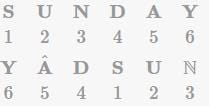RRB JE CE (CBT I) Mock Test- 1 - Question 41

Find the odd one out from the given alternatives.

Detailed Solution for RRB JE CE (CBT I) Mock Test- 1 - Question 41 Except in pairs 33- 56, in all others the sum of digits of one number is equal to the sum of digits of all the second number.
RRB JE CE (CBT I) Mock Test- 1 - Question 42

In the question below, there are few statements followed by few conclusions. You have to take the given statements to be true even if they seem to be at variance with commonly known facts and then decide which of the given conclusion logically follow(s) from the given statements.

Statements:

All horses are rivers.

All rivers are jungles.

No Jungle is flower.

Conclusions:

I. No horse is flower.

II. No flower is horse.

Detailed Solution for RRB JE CE (CBT I) Mock Test- 1 - Question 42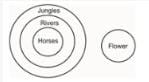If both Conclusions I and II follow.

RRB JE CE (CBT I) Mock Test- 1 - Question 43

Choose the missing terms out of the given alternatives.

ef_h_gfe_hef_ehgefg_

Detailed Solution for RRB JE CE (CBT I) Mock Test- 1 - Question 43 The given cyclic order series follows the pattern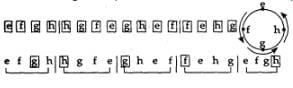RRB JE CE (CBT I) Mock Test- 1 - Question 44

What should come next in the following letter sequence:

A A B A B C A B C D A B C D E A B C D E F A B C D E F G A B C D E F G

Detailed Solution for RRB JE CE (CBT I) Mock Test- 1 - Question 44 The sequence is A AB ABC ABCD.........
RRB JE CE (CBT I) Mock Test- 1 - Question 45

In the given figure, circle represents youths, triangle represents the persons who are in job and rectangle represents illiterate person.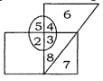Which of the following number depicts, illiterate youths who have job?

Detailed Solution for RRB JE CE (CBT I) Mock Test- 1 - Question 45 The number of illiterate youths and who have a job are three.
RRB JE CE (CBT I) Mock Test- 1 - Question 46

What comes in place of the question mark?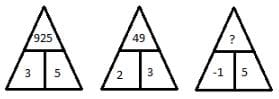Detailed Solution for RRB JE CE (CBT I) Mock Test- 1 - Question 46 The numbers obtained by squaring the numbers at the bottom are combined together physically to get the number in the upper part.
RRB JE CE (CBT I) Mock Test- 1 - Question 47

what comes in place of?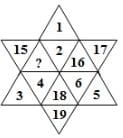Detailed Solution for RRB JE CE (CBT I) Mock Test- 1 - Question 47 The given figure contains numbers 1 to 6 in three alternate segments, the smaller number being towards the outside and the numbers 14 to 19 in the remaining three alternate segments with the smaller number towards the inside.
RRB JE CE (CBT I) Mock Test- 1 - Question 48

Find the water image of the object given in the question figure.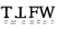Detailed Solution for RRB JE CE (CBT I) Mock Test- 1 - Question 48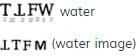RRB JE CE (CBT I) Mock Test- 1 - Question 49

A Piece of paper is folded and cut as shown below in the question figure. From the given answer figures, indicate how it will appear when opened.

Question figure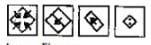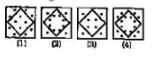Detailed Solution for RRB JE CE (CBT I) Mock Test- 1 - Question 49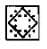Hence option 4 is correct answer.

RRB JE CE (CBT I) Mock Test- 1 - Question 50

In the following questions, four/five figures are given. Three/four are similar in a certain way and so form a group. Find out which one of the figures does not belong to that group.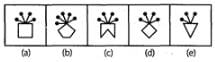Detailed Solution for RRB JE CE (CBT I) Mock Test- 1 - Question 50 In all other figures, the number of pins at the top of the figure are equal to the number of sides of the figure.
RRB JE CE (CBT I) Mock Test- 1 - Question 51

Which of the following words cannot be formed from 'GENERALIZATION'?

Detailed Solution for RRB JE CE (CBT I) Mock Test- 1 - Question 51 Using the letters of the given word, the word 'LIZARD' cannot be formed.
RRB JE CE (CBT I) Mock Test- 1 - Question 52

If ‘+’ stands for multiplication, ‘x’ stands for division, ‘–’ stands for addition and ‘÷’ stands for subtraction, what is the answer for the following equation?

20 – 5 ÷ 18 x (3 + 2) = ?

Detailed Solution for RRB JE CE (CBT I) Mock Test- 1 - Question 52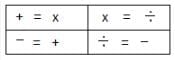Given expression is

20−5÷18×(3+2)=?

After conversions:

?=20+5−18÷(3×2)

or, ?=20+5−18+6

or, ?=20+5−3

Or.? = 25 - 3 = 22

RRB JE CE (CBT I) Mock Test- 1 - Question 53

The day on 18.09.1977 was Sunday. A couple was married on this date. In the next 15 years, there how many marriage anniversaries would fall on Sunday?

Detailed Solution for RRB JE CE (CBT I) Mock Test- 1 - Question 53 1977 is an ordinary year. We know that the calendar of an ordinary year repeats after 6 yrs or 11 yrs.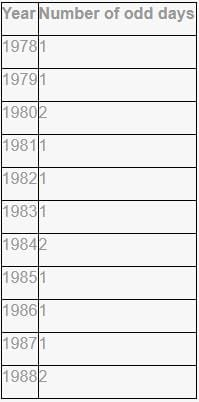Therefore,

Number of odd days from 18.09.1977 to 18.09.1983=7, i.e., 0 odd day

It means that in 1983, 18th September would fall on Sunday.

and number of odd days from 18.09.1977 to 18.09.1988 = 14, i.e., 0 odd day.

Now, it is clear that 2 marriage anniversaries would fall on Sunday in the next 15 yr.

RRB JE CE (CBT I) Mock Test- 1 - Question 54

In a row of girls, if Rohini who is 12th from the left and Mihika who is 9th from the right, if they interchange their seats then Rohini becomes 18th from the left. How many girls are there in the row?

Detailed Solution for RRB JE CE (CBT I) Mock Test- 1 - Question 54 When Rohini and Mihika interchange their places, then Rohini becomes on 18th place, it means Rohini who was on the 12th place comes at the 6th place after crossing 5 places. Therefore, there are 5 girls between Rohini and Mihika. So, the total number of the girls in the row = (18 + 9) -1 = 26 or (15 + 12) – 1 = 26.
RRB JE CE (CBT I) Mock Test- 1 - Question 55

Study the diagram given below and answer the question.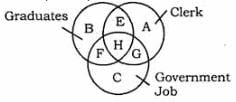Which part represents those clerks who are graduates and have government job?

Detailed Solution for RRB JE CE (CBT I) Mock Test- 1 - Question 55 'H' represents those clerks who are graduates and have government job.
RRB JE CE (CBT I) Mock Test- 1 - Question 56

Who was recently elected as the Deputy Chairman of the Rajya Sabha in the Parliament?

Detailed Solution for RRB JE CE (CBT I) Mock Test- 1 - Question 56 Harivansh Narayan Singh Rajya Sabha MP from Bihar since 2014 was recently elected as the new Deputy Chairman of Rajya Sabha in the Parliament.

Earlier in his career he was a journalist with 'Prabhat Khabar' and then was chosen as the media advisor to former Prime Minister Chandra Shekhar.

RRB JE CE (CBT I) Mock Test- 1 - Question 57

Recently, Ministry of External Affairs organised 11th World Hindi Conference at which of the following Indian ocean states?

Detailed Solution for RRB JE CE (CBT I) Mock Test- 1 - Question 57 11th edition of World Hindi Conference was organised in Swami Vivekanand International Convention Centre, Pailles Mauritius.

Theme of the Conference: "Hindi Vishwa Aur Bharatiy Sanskriti". It was organised by the Ministry of External Affairs, Government of India in association with the Government of Mauritius

RRB JE CE (CBT I) Mock Test- 1 - Question 58

Right to Information, an important legislative act to promote transparency & strengthen the democracy of India came into being on which year?

Detailed Solution for RRB JE CE (CBT I) Mock Test- 1 - Question 58 On October 12, 2005, Right To Information came into being after passage of an Act in the Indian parliament to bring in transparency in governmental procedures and end corruption

Using rtionline.gov.in citizens of India can seek information to turn India into a participatory democracy.

According to the government guidelines, 'Any person who desires to obtain information shall submit a written or electronic request in English or Hindi or in the official language of the area to the Central Public Information Officer or his/her counterpart at the state level.'

RRB JE CE (CBT I) Mock Test- 1 - Question 59

Which Indian state excelled to be the top performer in the recently released 'Ease of Living Index 2018' by Ministry of Housing & Urban Affairs?

Detailed Solution for RRB JE CE (CBT I) Mock Test- 1 - Question 59 Andhra Pradesh topped the charts among Indian States in terms of "Ease of Living Index 2018" rankings launched by the Ministry of Housing and Urban Affairs, followed by Odisha and Madhya Pradesh.

The Index seeks to assist states in undertaking a 360-degree assessment of their strengths, weaknesses, opportunities, and threat & innovate to perform in the governance, identity and culture, education, health, safety and security, economy, affordable housing, land use planning etc. for its residents.

RRB JE CE (CBT I) Mock Test- 1 - Question 60

Which of the following government entity started 'Seva Bhoj Yojna' - a scheme to reimburse central share of CGST and IGST on food, prasad, langar etc. offered by religious and charitable institutions?

Detailed Solution for RRB JE CE (CBT I) Mock Test- 1 - Question 60 Ministry of culture recently launched the Seva Bhoj Yojna, a scheme to reimburse central share of CGST and IGST on food, prasad, langar etc. offered by religious and charitable institutions.

Institutions like Temples, Gurudwara, Mosque, Church, Dharmik Ashram, Dargah etc. who are established and serving free food for more than 5 years to more than 5000 people every month without any discrimination are the eligible entities.

RRB JE CE (CBT I) Mock Test- 1 - Question 61

Google recently, unveiled ____________ which will quickly render any PDF with Indian language content into editable text using Artificial Intelligence.

Detailed Solution for RRB JE CE (CBT I) Mock Test- 1 - Question 61 During the 4th edition of 'Google for India' event, the Project Navlekha was unveiled which in Sanskrit means "a new way to write". It will quickly render any PDF with Indian language content into editable text using Artificial Intelligence.

Under the project, The registered publishers will be provided with free web hosting service along with a branded domain for 3 years and having AdSense support to help monetising their content.

RRB JE CE (CBT I) Mock Test- 1 - Question 62

Which of the following Indian states launched a scheme, 'Kanyashree', to incentivize adolescent girl child education to combat issue of child marriages?

Detailed Solution for RRB JE CE (CBT I) Mock Test- 1 - Question 62 Kanyashree was launched in 2013 by the West Bengal government to prevent child marriage in economically backward sections with an annual household income of ₹1.2 lakh.

Eligible girls got ₹1,000 a year scholarship from ages 13 to 18 years and a one-time grant of ₹25,000 on remaining unmarried till 18 years. More than 5 million girl children benefited from the scheme.

Recently the annual income limit was relaxed to any girl child within the age limit.

RRB JE CE (CBT I) Mock Test- 1 - Question 63

Which of the following government institution launched "Pitch to MOVE", a business opportunity platform for budding entrepreneurs?

Detailed Solution for RRB JE CE (CBT I) Mock Test- 1 - Question 63 NITI Aayog recently launched "Pitch to MOVE", a business opportunity platform for budding entrepreneurs to promote their business ideas.

It aims to identify and incentivise the start-ups, which will help the Government realize its vision of Shared, Connected, Intermodal and Environment Friendly Mobility for India.

The objective is to harness the latest disruption for generating employment and growth in our country

RRB JE CE (CBT I) Mock Test- 1 - Question 64

Ghoomar is a popular traditional folk dance from which region in India?

Detailed Solution for RRB JE CE (CBT I) Mock Test- 1 - Question 64 Ghoomar is a traditional dance from Rajasthan's Bhil tribe performed only by the women during celebrations pertaining to Goddess Saraswathi, Diwali, Holi etc.

Attire for women: Ghagras along with colourful chairs and heavy embroidery. Works garment.

RRB JE CE (CBT I) Mock Test- 1 - Question 65

Which of the following International Organisations are headquartered in Washington D.C., USA?

Detailed Solution for RRB JE CE (CBT I) Mock Test- 1 - Question 65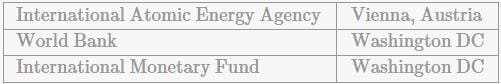RRB JE CE (CBT I) Mock Test- 1 - Question 66

Match the following trophies with their respective sports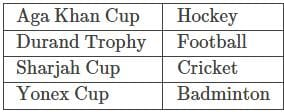Detailed Solution for RRB JE CE (CBT I) Mock Test- 1 - Question 66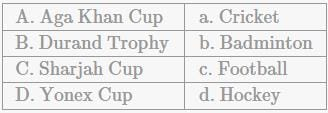RRB JE CE (CBT I) Mock Test- 1 - Question 67

Dhauladhar National Park is situated in which of the following Indian state?

Detailed Solution for RRB JE CE (CBT I) Mock Test- 1 - Question 67 Dhauladhar National Park is situated at a distance of 13kms from the city of Palampur in the Indian state of Himachal Pradesh.It is filled with exotic flora and fauna, and is home to different types of deer, red fox, sambhar, Asiatic lion, angora rabbits, black bears and leopards.It covers an area of 30 acres.
RRB JE CE (CBT I) Mock Test- 1 - Question 68

Daman & Diu is Union territory of India comprising 2 different areas separated by which of the following?

Detailed Solution for RRB JE CE (CBT I) Mock Test- 1 - Question 68 Arabian Sea separates the two areas of the union territory, Daman & Diu, bordered by the state of Gujarat.

With an area of only 112 square kms, it is the second smallest UT of India after Lakshadweep.

RRB JE CE (CBT I) Mock Test- 1 - Question 69

Recently, BOMBAY NATURAL HISTORY SOCIETY opened its regional centre at which of the following important Wetland in India?

Detailed Solution for RRB JE CE (CBT I) Mock Test- 1 - Question 69 Recently, BOMBAY NATURAL HISTORY SOCIETY opened its regional centre in the premises of The Wetland Research and Training Centre (WRTC) of the Chilika Development Authority (CDA) at Chandrapur in Chilika.

Here, BNHS would be engaged in identifying the air route of the migratory birds flocking the Chilika lake during winter & sample collection, training related to bird census among other important conservatory purposes.

RRB JE CE (CBT I) Mock Test- 1 - Question 70

With which of the following Financial Institution would you associate 'Prompt Corrective Action'?

Detailed Solution for RRB JE CE (CBT I) Mock Test- 1 - Question 70 The Reserve Bank of India has specified certain regulatory guidelines, as a part of prompt corrective action (PCA) Framework, in terms of 3 parameters,

- capital to risk weighted assets ratio (CRAR),

- net non-performing assets (NPA) and

- Return on Assets (RoA), applicable only to commercial banks and not extended to co-operative banks, non-banking financial companies (NBFCs) & other financial institutes

RRB JE CE (CBT I) Mock Test- 1 - Question 71

Which of the following rays exhibits the property of light wave?

Detailed Solution for RRB JE CE (CBT I) Mock Test- 1 - Question 71 Gamma rays, X-rays, microwaves and radio waves behave as a light wave and they exhibit properties such as reflection, refraction, diffraction etc.
RRB JE CE (CBT I) Mock Test- 1 - Question 72

A ball thrown vertically upwards with an initial velocity of 19.6 m/s returns in 4 seconds. Which one of the following is the maximum height attained by the ball?

Detailed Solution for RRB JE CE (CBT I) Mock Test- 1 - Question 72

Time taken by ball to the Highest point = 4 / 2

= 2 Second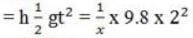= 19.6 m.

RRB JE CE (CBT I) Mock Test- 1 - Question 73

Which amongst the following is the most elastic?

Detailed Solution for RRB JE CE (CBT I) Mock Test- 1 - Question 73 Steel is the most elastic
RRB JE CE (CBT I) Mock Test- 1 - Question 74

A transformer works with

Detailed Solution for RRB JE CE (CBT I) Mock Test- 1 - Question 74 A transformer works with Alternating current.
RRB JE CE (CBT I) Mock Test- 1 - Question 75

The material having maximum ductility is:

Detailed Solution for RRB JE CE (CBT I) Mock Test- 1 - Question 75 The material having maximum ductility is Mild steel
RRB JE CE (CBT I) Mock Test- 1 - Question 76

Magnetic effect of current was discovered by

Detailed Solution for RRB JE CE (CBT I) Mock Test- 1 - Question 76 Magnetic effect of current was discovered by Oersted.
RRB JE CE (CBT I) Mock Test- 1 - Question 77

Modern communication systems use -

Detailed Solution for RRB JE CE (CBT I) Mock Test- 1 - Question 77 Modern communication systems use Digital circuits
RRB JE CE (CBT I) Mock Test- 1 - Question 78

Detailed Solution for RRB JE CE (CBT I) Mock Test- 1 - Question 78 Madam Curie was born in Poland.
RRB JE CE (CBT I) Mock Test- 1 - Question 79

When a body is immersed in a liquid, the force acting on it is?

Detailed Solution for RRB JE CE (CBT I) Mock Test- 1 - Question 79 According to the Principle of Flotation, when a body is immersed in a fluid, two forces act on it:

(1) The weight of the body acting vertically downward through the centre of gravity of the body, and

(2) The upthrust of the fluid acting vertically upward through the centre of gravity of the fluid displaced, i.e.. centre of buoyancy. The upthrust is also termed as buoyant force.

RRB JE CE (CBT I) Mock Test- 1 - Question 80

Which of the following is the best conductor of Electricity?

Detailed Solution for RRB JE CE (CBT I) Mock Test- 1 - Question 80 This is because salt water is a good conductor of electricity. Salt molecules are made of sodium ions and chlorine ions. (An ion is an atom that has an electrical charge because it has either gained or lost an electron.)
RRB JE CE (CBT I) Mock Test- 1 - Question 81

Mach number is used in connection with the speed of -

Detailed Solution for RRB JE CE (CBT I) Mock Test- 1 - Question 81 Mach number is used in connection with the speed of Aircraft.
RRB JE CE (CBT I) Mock Test- 1 - Question 82

Most soluble in water is —

Detailed Solution for RRB JE CE (CBT I) Mock Test- 1 - Question 82 Water is polar so any compound that is polar is soluble in water. Sugar (C12H22O11) is a polar covalent molecule. When it dissolves in water, the weak bonds between the carbon, hydrogen and oxygen atoms are broken.
RRB JE CE (CBT I) Mock Test- 1 - Question 83

If linear momentum is increased by 50%, the kinetic energy will increase by

Detailed Solution for RRB JE CE (CBT I) Mock Test- 1 - Question 83 If linear momentum is increased by 50%, the kinetic energy will increase by 125%
RRB JE CE (CBT I) Mock Test- 1 - Question 84

Which of the following is not a semiconductor?

Detailed Solution for RRB JE CE (CBT I) Mock Test- 1 - Question 84 Arsenic is not a semiconductor.
RRB JE CE (CBT I) Mock Test- 1 - Question 85

Which of the following colour will scatter the most while passing through a prism?

Detailed Solution for RRB JE CE (CBT I) Mock Test- 1 - Question 85 Lights of different colour depending upon their scattering in ascending ordor are violet, indigo, blue, green, yellow, orange and red.
RRB JE CE (CBT I) Mock Test- 1 - Question 86

Brass gets discoloured in air due to the presence of which gas in air ?

Detailed Solution for RRB JE CE (CBT I) Mock Test- 1 - Question 86 Hydrogen sulphide can corrode metals such as iron, steel, copper, and brass, tarnish silverware, discolour copper and brass utensils, cause yellow or black stains on fixtures and alter the appearance and taste of cooked foods.
RRB JE CE (CBT I) Mock Test- 1 - Question 87

Bleaching powder is made from —

Detailed Solution for RRB JE CE (CBT I) Mock Test- 1 - Question 87 A mixture with lime and calcium chloride, it is marketed as chlorine powder or bleaching powder for water treatment and as a bleaching agent.
RRB JE CE (CBT I) Mock Test- 1 - Question 88

At high altitudes the boiling point of water reduces because -

Detailed Solution for RRB JE CE (CBT I) Mock Test- 1 - Question 88 As we go to higher altitudes the atmospheric pressure reduces, which actually lowers the boiling point of water.
RRB JE CE (CBT I) Mock Test- 1 - Question 89

The point, through which the whole weight of the body acts, irrespective of its position, is known as

Detailed Solution for RRB JE CE (CBT I) Mock Test- 1 - Question 89 The point, through which the whole weight of the body acts, irrespective of its position, is known as centre of gravity.
RRB JE CE (CBT I) Mock Test- 1 - Question 90

Vitamin A is also known as ?

Detailed Solution for RRB JE CE (CBT I) Mock Test- 1 - Question 90 Retinol: Retinol is an active form of vitamin A. It produces the pigments in the retina of the eye.
RRB JE CE (CBT I) Mock Test- 1 - Question 91

Avogadro constant, NA is the number of molecules in one mole of a substance and its value (NA) is

Detailed Solution for RRB JE CE (CBT I) Mock Test- 1 - Question 91 Avogadro constant, NA is the number of molecules in one mole of a substance and its value (NA) is 6.02 × 1023 mol
RRB JE CE (CBT I) Mock Test- 1 - Question 92

Ginger is an example of –

Detailed Solution for RRB JE CE (CBT I) Mock Test- 1 - Question 92 Ginger is a rhizome (modified plant stem).
RRB JE CE (CBT I) Mock Test- 1 - Question 93

When CaC2 is heated in atmospheric nitrogen in an electric furnance the compound formed is ________.

Detailed Solution for RRB JE CE (CBT I) Mock Test- 1 - Question 93 Solubility of alkali metal hydroxide increases as the size of the alkali metal increases.
RRB JE CE (CBT I) Mock Test- 1 - Question 94

Brass is an alloy of —

Detailed Solution for RRB JE CE (CBT I) Mock Test- 1 - Question 94 Zinc and Copper: Brass is a metallic alloy that is made of copper and zinc.
RRB JE CE (CBT I) Mock Test- 1 - Question 95

Dioptre is the unit of:

Detailed Solution for RRB JE CE (CBT I) Mock Test- 1 - Question 95 Dioptre is the unit of Power of a lens.
RRB JE CE (CBT I) Mock Test- 1 - Question 96

In which of the following blocks of the periodic table are the Alkali & Alkaline earth metals included?

Detailed Solution for RRB JE CE (CBT I) Mock Test- 1 - Question 96 The alkali and alkaline earth metals are included in the s-block of the periodic table.
RRB JE CE (CBT I) Mock Test- 1 - Question 97

Cockroach is a:

Detailed Solution for RRB JE CE (CBT I) Mock Test- 1 - Question 97 Cockroaches are omnivores and can eat almost any type of organic material in order to survive, which makes them one of the healthiest insects on the planet. Although they prefer sweets, meats and starches, they are also known to consume other items such as hair, books and decaying matter.
RRB JE CE (CBT I) Mock Test- 1 - Question 98

Permanent hardness of water may be removed by the addition of ____.

Detailed Solution for RRB JE CE (CBT I) Mock Test- 1 - Question 98 Sodium carbonate, Na2CO3, is also known as washing soda. It can remove temporary and permanent hardness from water.
RRB JE CE (CBT I) Mock Test- 1 - Question 99

A person can jump higher on the moon's surface than on the earth because:

Detailed Solution for RRB JE CE (CBT I) Mock Test- 1 - Question 99 The acceleration due to gravity in moon is smaller than that on the earth.
RRB JE CE (CBT I) Mock Test- 1 - Question 100

When something is dropped it does not fall with uniform ____.

Detailed Solution for RRB JE CE (CBT I) Mock Test- 1 - Question 100 When something is dropped it does not fall with uniform velocity. They are under the constant acceleration of the gravitational force.

## RRB JE Mock Test Series for Civil Engineering (CE)

158 tests
 Use Code STAYHOME200 and get INR 200 additional OFF Use Coupon Code
Information about RRB JE CE (CBT I) Mock Test- 1 Page
In this test you can find the Exam questions for RRB JE CE (CBT I) Mock Test- 1 solved & explained in the simplest way possible. Besides giving Questions and answers for RRB JE CE (CBT I) Mock Test- 1, EduRev gives you an ample number of Online tests for practice

158 tests